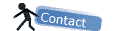Image Calculator...
Performs arithmetic and logical operations between two images selected from popup menus. Image1 or both the Image1 and Image2 can be stacks. If both are stacks, they must have the same number of slices. Image1 and Image2 must be the same data type but do not have to be the same size.You can select one of 8 operators from the Operation: popup menu. Check Create New Window and a new image or stack will be created to hold the result. Otherwise, the result of the operation replaces some or all of Image1. With 32-bit (float) images, pixels resulting from division by zero are set to the largest possible value (3.4e38). This value can be redefined in Edit/Options/Miscellaneous/Divide by Zero Value.

 AND img1= img1 AND img2 OR img1 = img1 OR img2 XOR img1 = img1 XOR img2 Min img1 = min(img1,img2) Max img1 = max(img1,img2) Average img1 = (img1+img2)/2 Difference img1 = |img1-img2| Copy img1 = img2

Some examples with two images:result with "AND":result with "OR":result with "XOR":result with "Difference":Single Image Operators>

The commands in this submenu add (subtract, multiply, etc.) a constant to each pixel in the active image or selection. When the result value overflows/underflows the legal range of the image's data type, the value is reset to the maximum/minimum value. With stacks, selections are ignored and the specified operation is performed on all slices in the stack.

Adds a constant to the image. With 8-bit images, results greater than 255 are set to 255. With 16-bit signed images, results greater than 65,535 are set to 65,535.

Subtract...
Subtracts a constant from the image. With 8-bit and 16-bit images, results less than 0 are set to 0.

Multiply...
Multiplies the image by the specified real constant. With 8-bit images, results greater than 255 are set to 255. With 16-bit signed images, results greater than 65,535 are set to 65,535.

Divide...
Divides the image by the specified real constant. Attempts to divide by zero will be ignored.

AND...
Does a bitwise AND of the image and the specified binary constant.

OR...
Does a bitwise OR of the image and the specified binary constant.

XOR...
Does a bitwise XOR of the image and the specified binary constant.

Min...
Pixels in the image with a value less than the specified constant are replaced by the constant.

Max...
Pixels in the image with a value greater than the specified constant are replaced by the constant.

Gamma...
Applies the function f(p) = (p/255)^gamma*255 to each pixel (p) in the image or selection, where 0.1 <= gamma <= 5.0. For RGB images, this function is applied to all three colour channels. For 16-bit images, the image min and max are used for scaling instead of 255.

Log...
For 8-bit images, applies the function f(p) = log(p) * 255/log(255) to each pixel (p) in the image or selection. For RGB images, this function is applied to all three colour channels. For 16-bit images, the image min and max are used for scaling instead of 255. For float images, no scaling is done. To calculate log10 of the image, multiply the result of this operation by 0.4343 (1/log(10).

Reciprocal
Generates the reciprocal of the active image or selection. Only works with 32-bit float images.

Rotate>

This submenu contains commands that rotate the active image or stack.
Here the original image :Flip Vertical
Turns the image or selection upside down.Flip Horizontal
Replaces the image or selection with a mirror image of the original.Rotate 90 Degrees Right
Rotates the entire image or stack clockwise 90 degrees.Rotate 90 Degrees Left
Rotates the entire image or stack counter-clockwise 90 degrees.Arbitrarily...
Use this dialog to rotate the active image or selection clockwise the specified number of degrees. Check Interpolate to use bilinear interpolation.Translation>
Translate the active image or stack.The values of translation on X and Y are specified in the popup menus.